# Expression of a variable from the formula + The Law of Cosines - math problems

#### Number of problems found: 19

• Two forces 3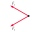Two forces of magnitude 8 Newtons and 15 Newtons respectively act at a point. If the resultant force is 17 Newtons, find the angle between the forces.
• Calculate triangleIn the triangle ABC, calculate the sizes of all heights, angles, perimeters and its area, if given a-40cm, b-57cm, c-59cm
• The farmer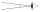The farmer sees the back fence of the land, which is 50 m long at a viewing angle of 30 degrees. It is 92 m away from one end of the fence. How far is it from the other end of the fence?
• Triangle's centroid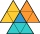In the triangle ABC the given lengths of its medians tc = 9, ta = 6. Let T be the intersection of the medians (triangle's centroid) and point is S the center of the side BC. The magnitude of the CTS angle is 60°. Calculate the length of the BC side to 2 d
• Distance of pointsA regular quadrilateral pyramid ABCDV is given, in which edge AB = a = 4 cm and height v = 8 cm. Let S be the center of the CV. Find the distance of points A and S.What is the volume of a quadrilateral oblique prism with base edges of length a = 1m, b = 1.1m, c = 1.2m, d = 0.7m, if a side edge of length h = 3.9m has a deviation from the base of 20° 35' and the edges a, b form an angle of 50.5°.
• Two chordsFrom the point on the circle with a diameter of 8 cm, two identical chords are led, which form an angle of 60°. Calculate the length of these chords.
• Viewing angleThe observer sees a straight fence 60 m long at a viewing angle of 30°. It is 102 m away from one end of the enclosure. How far is the observer from the other end of the enclosure?
• Triangle from medianCalculate the perimeter, content, and magnitudes of the triangle ABC's remaining angles, given: a = 8.4; β = 105° 35 '; and median ta = 12.5.
• Two grovesTwo groves A, B are separated by a forest, both are visible from the hunting grove C, which is connected to both by direct roads. What will be the length of the projected road from A to B, if AC = 5004 m, BC = 2600 m and angle ABC = 53° 45 ’?
• The spacecraft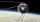The spacecraft spotted a radar device at altitude angle alpha = 34 degrees 37 minutes and had a distance of u = 615km from Earth's observation point. Calculate the distance d of the spacecraft from Earth at the moment of observation. Earth is considered a
• Circular railwayThe railway connects in a circular arc the points A, B, and C, whose distances are | AB | = 30 km, AC = 95 km, BC | = 70 km. How long will the track from A to C?
• A rhombusA rhombus has sides of length 10 cm, and the angle between two adjacent sides is 76 degrees. Find the length of the longer diagonal of the rhombus.
• Parallelogram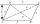The sides of the parallelogram are 8 cm and 6 cm long, and the diagonals' angle is 60°. What is its area?
• Children playgroundThe playground has a trapezoid shape, and the parallel sides have a length of 36 m and 21 m. The remaining two sides are 14 m long and 16 m long. Find the size of the inner trapezoid angles.
• Calculate 2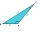Calculate the largest angle of the triangle whose side are 5.2cm, 3.6cm, and 2.1cm
• The pondWe can see the pond at an angle 65°37'. Its end points are 155 m and 177 m away from the observer. What is the width of the pond?
• Largest angle of the triangleCalculate the largest angle of the triangle whose sides have the sizes: 2a, 3/2a, 3a
• Heron backlawCalculate missing side in a triangle with sides 25 and 13 and area 152.

Do you have an exciting math question or word problem that you can't solve? Ask a question or post a math problem, and we can try to solve it.

We will send a solution to your e-mail address. Solved examples are also published here. Please enter the e-mail correctly and check whether you don't have a full mailbox.

Cosine rule uses trigonometric SAS triangle calculator. Expression of a variable from the formula - math problems. The Law of Cosines - math problems.